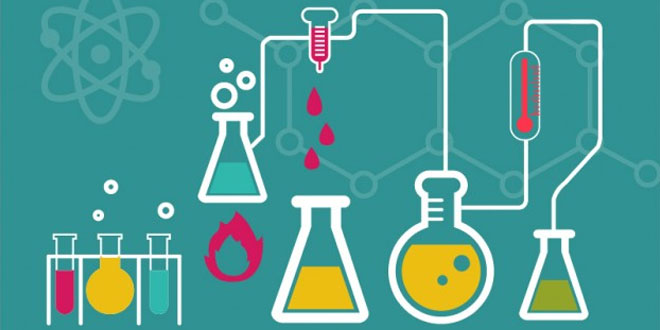Saturday , November 28 2020# 11th CBSE NCERT Chemistry Mid Term Exam Set B (2019-20)

Time: 3 Hrs.
Marks: 70
Class: 11th
Date: 13/09/2019

## General Instructions:

1. All questions are compulsory (11th CBSE NCERT Chemistry Mid Term Exam).
2. Q’s 1 to 20 are of 1 mark each.
3. Q’s 21 to 27 are of 2 marks each.
4. Q’s 28 to 34 are of 4 marks each.
5. Q’s 35 to 37 are of 5 marks each.
6. All questions are compulsory.

### Section A

#### Question: (i) In which one of the following pair the two species are isoelectronic

1. 39K+ and 40Ar
2. 23Na and 23Na+
3. 40Ca²+ and 39K
4. 23Na and 24Mg²+

#### Question: (ii). The no. of atoms in 0.1 ml of triatomic gas is

1. 1.800 × 10²²
2. 1.806 × 10²³
3. 6.026 × 10²²
4. 3.600 × 10²²

#### Question: (iii). Which of the following concentration term are dependent of temperature

1. Molality only
2. Molality and Mole fraction
3. Molarity and Normality
4. Molarity only

#### Question: (iv). The maximum no. of molecule is present in

1. 5l of N2 gas at STP
2. 10 g of O2 gas
3. 0.5 g of H2 gas
4. 15 l of H2 gas at STP

#### Question: (v) Which of the following sets of quantum numbers are not possible:

1. n = 3, l = 0, m = 0, S = +½
2. n = 3, l = 0, m = +10, S = -½
3. n = 3, l = 2, m = 0, S = -½

1. 2, 0
2. 0, 2
3. 1, 2
4. 2, 1

#### Question: (vii) The electron identified by quantum numbers n and l are:

1. n = 4, l = 1
2. n = 4, l = 0
3. n = 3, l = 2
4. n = 3, l = 1

1. 4<2<3<1
2. 2<4<1<3
3. 1<3<2<4
4. 3<4<2<1

1. n
2. 2n
3. n²
4. 2

1. Na<K<Rb
2. I+ <I<I
3. Fe²+<Fe³+Fe
4. F<O<N

#### Question: (x) Which of the following properly increases along a period (in general)

1. Atomic size
2. Ionization enthalpy
3. electron gain enthalpy
4. Ionic size

1. N
2. O
3. S
4. Br

1. s>p>d>f
2. s<p<d<f
3. s>p>d<f
4. s<p<d>f

1. C2H6
2. B2H6
3. SiH4
4. PH23

1. SF4
2. BrF5
3. SO2
4. PH2

1. H2O
2. NH3
3. CO2
4. CH4

1. SP²
2. SP³
3. SP
4. dSP²

1. 1.987
2. 8.31 × 107
3. 0.082
4. 8.314

#### Question: (xviii) The compressibility factor for an ideal gas is:

1. Unity at all temperature
2. Unity at Boyle’s temperature
3. Zero
4. decrease with pressure

1. V-b
2. (RT)-1
3. (p + a/v2)
4. RT

#### Question: (xx) The ratio of partial pressure of gaseous component to the total vapour pressure of the mixture is equal to:

1. mass of the component
2. molefraction of the component
3. mass % of the component
4. molecular mass

### Section B: 11th CBSE NCERT Chemistry Mid Term Exam

#### Question: (xxi)

1. State law of multiple proportion with suitable example.
2. What is limiting reagent.

1. CO32-
2. O3

#### Question: (xxvii)

1. Why noble gas have the largest positive electron gain enthalpy in their respective periods.
2. Explain why chlorine can be converted into chloride ion more easily as compared to fluoride ion.

### Section C

#### Question: (xxviii)

1. From the elements: Cl, Br, F, O, Al, C, Li, Cs and Xe Choose the following:
(a) the element with six electron in the valence shell.
(b) the element which belong to zero group.
(c) The  element which form large no. of compound
2. Out of Li and Be, which is expected to have second ionization enthalpy?

#### The reaction 2C + O2  →   2CO is carried out by taking 24g of C and 96g of O2. Find out

1. Which reactant is left in excess.
2. How much of it is left
3. How many gram of other reactant should be taken so that nothing is left at the end of the reaction.

#### 11th CBSE NCERT Chemistry Mid Term Exam – Question: (xxxi)

1. Explain the following term:
(a) Pauli exclusion principle
(b) Heisenberg Uncertainty Principal
2. Draw the shape of the orbital in which
n = 3, l = 2, m2 = -2

#### Question: (xxxii)

1. Write the resonating structure for SO3
2. Arrange the bond in order of increasing ionic character in the molecule LiF, K2O, N2, SO2, ClF3
3. What is significance of plus and minus sign in representing the orbital.

### Section D

#### Question: (xxxv)

1. How many unpaired electrons are present in SC(Scandium).
2. In a photo electric effect experiment, irradiation of a metal with a light of frequency 5.2 × 10-19J. Calculate threshold frequency (Vo) of the metal.

OR

1. Write the values of all the four quantum numbers of the last electron of calcium (Z = 20).
2. Explain why chromium has configuration 3d54S¹ and not 3d44S²
3. In the building of the atom, the filling of 4S-Orbital take place before 3d orbital.

#### Question: (xxxvi)

1. Differentiate between bonding and Anti-bonding Molecular Orbitals.
2. Calculate the bond order and magnetic properties of O22- and O2on the basis of Molecular orbital theory.

#### OR

1. Define Hybridisation.
2. What are the conditions of Hybridisation.
3. Discuss the orbital structure of NH3 on the basis of Hybridisation.

#### Calculate the MF of the compound assuming that the whole of Hydrogen in the compound is present in water of crystallization. Molecular mass of the compound is 322.

1. Define atomic mass unit

OR

1. What are real gases. Discuss the deviation of real gas from ideal behavior?
2. State and explain the Dalton’s law of partial pressure.

## Light: 7th Class NCERT CBSE Science Chapter 15

Light 7th Class NCERT CBSE Science Chapter 15 Question: Briefly mention whether mirror change the direction of …